# Fraction calculator

The calculator performs basic and advanced operations with fractions, expressions with fractions combined with integers, decimals, and mixed numbers. It also shows detailed step-by-step information about the fraction calculation procedure. Solve problems with two, three, or more fractions and numbers in one expression.

## Result:

### 2/3 - 3/4 = -1/12 ≅ -0.08333333

Spelled result in words is minus one twelfth.

### How do you solve fractions step by step?

1. Subtract: 2/3 - 3/4 = 2 · 4/3 · 4 - 3 · 3/4 · 3 = 8/12 - 9/12 = 8 - 9/12 = -1/12
For adding, subtracting, and comparing fractions, it is suitable to adjust both fractions to a common (equal, identical) denominator. The common denominator you can calculate as the least common multiple of both denominators - LCM(3, 4) = 12. In practice, it is enough to find the common denominator (not necessarily the lowest) by multiplying the denominators: 3 × 4 = 12. In the following intermediate step, the fraction result cannot be further simplified by canceling.
In other words - two thirds minus three quarters = minus one twelfth.

#### Rules for expressions with fractions:

Fractions - simply use a forward slash between the numerator and denominator, i.e., for five-hundredths, enter 5/100. If you are using mixed numbers, be sure to leave a single space between the whole and fraction part.
The slash separates the numerator (number above a fraction line) and denominator (number below).

Mixed numerals (mixed fractions or mixed numbers) write as integer separated by one space and fraction i.e., 1 2/3 (having the same sign). An example of a negative mixed fraction: -5 1/2.
Because slash is both signs for fraction line and division, we recommended use colon (:) as the operator of division fractions i.e., 1/2 : 3.

Decimals (decimal numbers) enter with a decimal point . and they are automatically converted to fractions - i.e. 1.45.

The colon : and slash / is the symbol of division. Can be used to divide mixed numbers 1 2/3 : 4 3/8 or can be used for write complex fractions i.e. 1/2 : 1/3.
An asterisk * or × is the symbol for multiplication.
Plus + is addition, minus sign - is subtraction and ()[] is mathematical parentheses.
The exponentiation/power symbol is ^ - for example: (7/8-4/5)^2 = (7/8-4/5)2

#### Examples:

subtracting fractions: 2/3 - 1/2
multiplying fractions: 7/8 * 3/9
dividing Fractions: 1/2 : 3/4
exponentiation of fraction: 3/5^3
fractional exponents: 16 ^ 1/2
adding fractions and mixed numbers: 8/5 + 6 2/7
dividing integer and fraction: 5 ÷ 1/2
complex fractions: 5/8 : 2 2/3
decimal to fraction: 0.625
Fraction to Decimal: 1/4
Fraction to Percent: 1/8 %
comparing fractions: 1/4 2/3
multiplying a fraction by a whole number: 6 * 3/4
square root of a fraction: sqrt(1/16)
reducing or simplifying the fraction (simplification) - dividing the numerator and denominator of a fraction by the same non-zero number - equivalent fraction: 4/22
expression with brackets: 1/3 * (1/2 - 3 3/8)
compound fraction: 3/4 of 5/7
fractions multiple: 2/3 of 3/5
divide to find the quotient: 3/5 ÷ 2/3

The calculator follows well-known rules for order of operations. The most common mnemonics for remembering this order of operations are:
PEMDAS - Parentheses, Exponents, Multiplication, Division, Addition, Subtraction.
BEDMAS - Brackets, Exponents, Division, Multiplication, Addition, Subtraction
BODMAS - Brackets, Of or Order, Division, Multiplication, Addition, Subtraction.
GEMDAS - Grouping Symbols - brackets (){}, Exponents, Multiplication, Division, Addition, Subtraction.
Be careful, always do multiplication and division before addition and subtraction. Some operators (+ and -) and (* and /) has the same priority and then must evaluate from left to right.

## Fractions in word problems:About 6/9 of the sixth- grade pupils will be going to the parents' seminar. If 1/6 of the participants are girls, what part of the portion of sixth graders are boys?
• Leo hiked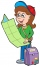Leo hiked 6/7 of a kilometer. Jericho hiked 2/3 kilometer. Who covered a longer distance? How much longer?
• Bucket of clay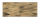Tina and Bill share a 12-ounce bucket of clay. By the end of the week, Tina has used 1/6 of the bucket, and Bill has used 2/3 of the bucket of clay. How many ounces are left in the bucket?
• Benhur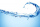Benhur boiled 1 1/4 liters of water in a kettle. After 10 1/2 minutes he measured the water again. It had 3/4 liters left in the kettle. What is the amount of water that evaporates every minutes?
• Ali bought 2Ali bought 5/6 litre of milk. He drank 1/2 litre and his brother drank 1/6 litre. How much litre of milk left?
• Translate 2Translate the given phrases to mathematical phrases. Thrice the sum of three fifths and two thirds less one half is what number?
• Mixed numbers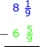Five and two-thirds minus 2 and one-half equals what number? A three and one-sixth B three and two-thirds C three and one-half D three and five-sixths
• Equation with mixed 2A number, X, is subtracted from 8 1/4. The result is 12 3/5. What is the value of X?
• SundarSundar has 50 chocolates. He gave 2/5 of these chocolates to Ram and he ate 1/5 of them. How many chocolates are left with Sundar?
• Mother 7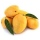Mother bought 18 fruits. 1/3 were pineapple and the rest  were mangoes . how many were mangoes
• Hotel 4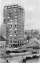A 360 room hotel has 1/3 of its rooms occupied at present. How many rooms are empty?
• PoundsThree pounds subtract 1/3 of a pound.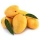A basket contains three types of fruits weighing 87/4 kg in all. If 23/4 kilograms of these are oranges, 48/7 kg are mangoes, and the rest are apples. What is the weight of the apples in the basket?